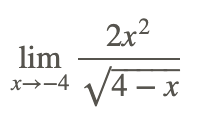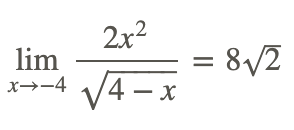# 1.6: Properties of Limit

•• Contributed by CK12
• CK12

You're familiar with the idea of a limit of a function, and that some limits are computed using numerical and graphical methods. Limits can also be evaluated using the properties of limits. How would you findwithout using a graph or using a table of values?

## Properties of Limits

Let’s begin with some observations about limits of some simple functions. Consider the following limit problems:We note that each of these functions is defined for all real numbers. If we apply our intuition for finding the limits we conclude correctly that:The above results can be encapsulated in the following limit properties:

Basic Limit Properties:Many functions can be expressed as the sums, differences, products, quotients, powers and roots of other more simple functions. The following properties are also useful in evaluating limits:

More Basic Limit Properties:Knowing these properties, allows evaluation of the limits of a wide range of functions.

Take the problem:Based on the properties above, the limit can be evaluated in the following steps:Therefore:Note that the application of the basic limit properties results, in this case, in a limit value that is the same as direct substitution of x=2 in the function.

Now, evaluateBased on the properties of limits, the limit can be evaluated in the following steps:Therefore:Again, note that the application of the basic limit properties results, in this case, in a limit value that is the same as direct substitution of x=2 in the function.

## Examples

### Example 1

Earlier, you were asked to findwithout using a graph or a table of values. This is a case where direct substitution to evaluate the limit gives the indeterminate form 0/0. But, reducing the fraction and then applying the basic limit properties above, we can evaluate the limit:### Example 2

Evaluatewhere f(x) is the rational function### Example 3

Find the following limit if it exists:Let’s apply the basic quotient rule to evaluate this limit.Therefore:Again, the limit result is the same as using direct substitution of x=−4 in the function.

## Review